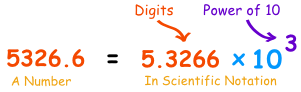# what is standard form?

THE SIMPLIFIED FORM OF A FRACTION IS CALLED ITS STANDARD FORM

## Standard Form of a Decimal Number

In Britain this is another name for Scientific Notation, where you write down a number this way:In this example, 5326.6 is written as 5.3266 × 103, because 5326.6 = 5.3266 × 1000 = 5326.6 × 103

In other Countries it just means "not in expanded form":

 561 500 + 60 + 1 Standard Form Expanded Form

• 3

THE SIMPLIFIED FORM OF A FRACTION IS CALLED ITS STANDARD FORM.

For example-standard form of 25/30=5/6.

• 2

thank you to answer my question.

• -1
What are you looking for?